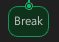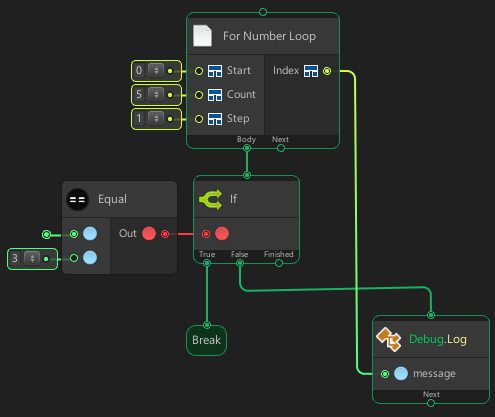# BreakThe Break node terminates the closest enclosing loop or switch statement in which it appears. Control is passed to the statement that follows the terminated statement, if any.

### Examples

In this example, the For Number Loop node is called at start. It then loop 5x each loop it call “if node” if the index is equal to 3 then it will stop the loop. The “Debug Log” node will called after “If node” finished whatever the condition is true or false, but when the index is 3 the node will never be called again because the loop is stopped by “Break” node.

Flow Graph:Generated Script:

```using UnityEngine;
using System.Collections.Generic;

public class Program : MonoBehaviour {
private int index;

public void Start() {
for(index = 0; index < 5; index += 1) {
if((index == 3)) {
break;
}
Debug.Log(index);
}
}
}```

Output:

```0
1
2```